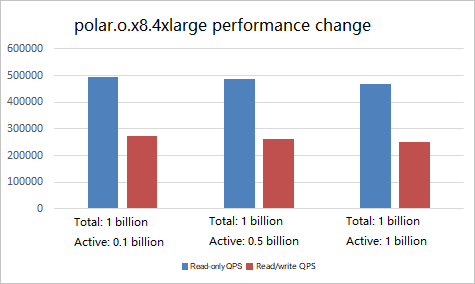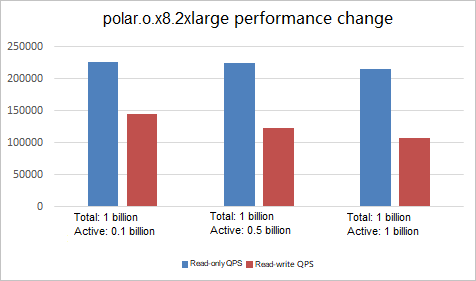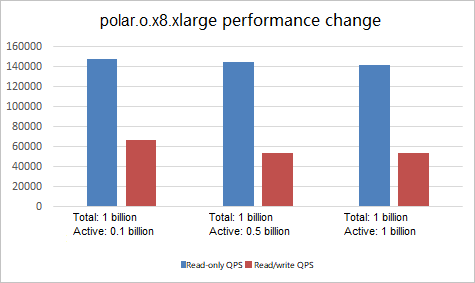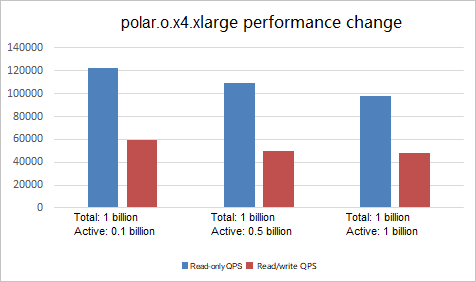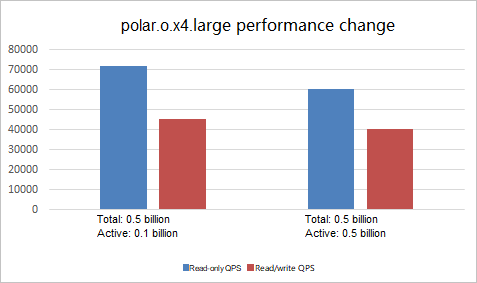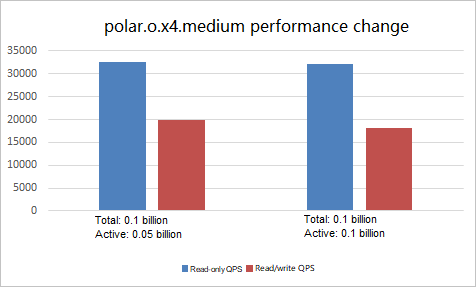This topic describes how to use pgbench to test the peak performance of the primary node in a PolarDB-O.

pgbench is a lightweight stress testing tool provided by PostgreSQL for running benchmark tests on PostgreSQL (compatible with Oracle). It executes the same sequence of SQL statements over and over, possibly in multiple concurrent database sessions.

## Test environment

• All tests are completed locally by using IP addresses and ports.
• ECS instance type: ecs.g5.16xlarge (64 cores, 256 GiB).
• Network type: VPC
• Operating system: 64-bit CentOS 7.6
Note CentOS 6 does not support PostgreSQL 11.

## Test metrics

• Read-only queries per second (QPS)

The number of SELECT statements executed per second when read-only operations are performed on the database.

The number of INSERT, SELECT, and UPDATE statements executed per second when read/write operations are performed on the database.

## Preparations

• Install PostgreSQL 11.
Run the following commands to install PostgreSQL 11 on your ECS instance:
``````yum install -y https://dl.fedoraproject.org/pub/epel/epel-release-latest-7.noarch.rpm
yum install -y postgresql11*
su - postgres
vi .bash_profile
export PS1="\$USER@`/bin/hostname -s`-> "
export LANG=en_US.utf8
export PGHOME=/usr/pgsql-11
export LD_LIBRARY_PATH=\$PGHOME/lib:/lib64:/usr/lib64:/usr/local/lib64:/lib:/usr/lib:/usr/local/lib:\$LD_LIBRARY_PATH
export DATE=`date +"%Y%m%d%H%M"`
export PATH=\$PGHOME/bin:\$PATH:.
export MANPATH=\$PGHOME/share/man:\$MANPATH
alias rm='rm -i'
alias ll='ls -lh'
unalias vi``````
• Edit parameters for your PolarDB cluster.
You must open a ticket to modify the YAML configuration file of your PolarDB cluster because some parameters cannot be edited in the Alibaba Cloud Management Console. The configurations are as follows:
``````default_statistics_target: "100"
max_wal_size: "64GB" #half mem size
effective_cache_size: "96GB" #3/4 mem size
max_parallel_workers_per_gather: "16" #half cpu core number
maintenance_work_mem: "2GB" #1/32 mem, don't exceed 8GB
checkpoint_completion_target: "0.9"
max_parallel_workers: "32" #cpu core number,don't exceed 64
max_prepared_transactions: "2100"
archive_mode: "off"
work_mem: "64MB" #mem 1/2000,don't exceed 128MB
wal_buffers: "16MB"
min_wal_size: "64GB" #1/4 mem size, min size 3GB (3 wal files, 2 as preallocated)
shared_buffers: "192GB" #75% mem size 8GB
max_connections: "12900"
polar_bulk_extend_size: "4MB"
polar_xlog_record_buffers: "25GB" #10~15% mem size,min size 1GB
hot_standby_feedback: "on"
full_page_writes: "off"
synchronous_commit: "on"
polar_enable_async_pwrite: "off"
polar_parallel_bgwriter_delay: "10ms"
polar_max_non_super_conns: '12800'
polar_parallel_new_bgwriter_threshold_lag: "6GB"
polar_use_statistical_relpages: "on"
polar_vfs.enable_file_size_cache: "on"``````
Note The preceding code snippet is used as an example to modify the configuration file of a 32-core 256-GB polar.o.x8.4xlarge cluster. Set the same memory size for the PolarDB cluster and an RDS for PostgreSQL cluster to compare their performance.

After the configuration file is modified, restart the PolarDB cluster to make the configuration take effect.

## Test procedure

1. Initialize test data based on the size of the target database.
• To initialize 5 billion data records, run the `pgbench -i -s 50000` command.
• To initialize 1 billion data records, run the `pgbench -i -s 10000` command.
• To initialize 500 million data records, run the `pgbench -i -s 5000` command.
• To initialize 100 million data records, run the `pgbench -i -s 1000` command.
2. Run the following commands to set environment variables:
``````export PGHOST=<The VPC-facing endpoint of the primary node in the PolarDB-O>
export PGPORT=<The VPC-facing port of the primary node in the PolarDB-O>
export PGDATABASE=postgres
export PGUSER=<The username to log on to the PolarDB-O>
• Create a script file named ro.sql to test read-only operations:
``````\set aid random_gaussian(1, :range, 10.0)
SELECT abalance FROM pgbench_accounts WHERE aid = :aid;``````
• Create a script file named rw.sql to test read/write operations:
``````\set aid random_gaussian(1, :range, 10.0)
\set bid random(1, 1 * :scale)
\set tid random(1, 10 * :scale)
\set delta random(-5000, 5000)
BEGIN;
UPDATE pgbench_accounts SET abalance = abalance + :delta WHERE aid = :aid;
SELECT abalance FROM pgbench_accounts WHERE aid = :aid;
UPDATE pgbench_tellers SET tbalance = tbalance + :delta WHERE tid = :tid;
UPDATE pgbench_branches SET bbalance = bbalance + :delta WHERE bid = :bid;
INSERT INTO pgbench_history (tid, bid, aid, delta, mtime) VALUES (:tid, :bid, :aid, :delta, CURRENT_TIMESTAMP);
END;``````
4. Perform the tests.
• Run the following commands to test read-only operations:
``````polar.o.x8.4xlarge, the total number of data records is 1 billion, the number of hot data records is 100 million.
pgbench -M prepared -v -r -P 1 -f ./ro.sql -c 128 -j 128 -T 120 -D scale=10000 -D range=100000000
polar.o.x8.4xlarge, the total number of data records is 1 billion, the number of hot data records is 500 million.
pgbench -M prepared -n -r -P 1 -f ./ro.sql -c 128 -j 128 -T 120 -D scale=10000 -D range=500000000
polar.o.x8.4xlarge, the total number of data records is 1 billion, the number of hot data records is 1 billion.
pgbench -M prepared -n -r -P 1 -f ./ro.sql -c 128 -j 128 -T 120 -D scale=10000 -D range=1000000000
polar.o.x8.2xlarge, the total number of data records is 1 billion, the number of hot data records is 100 million.
pgbench -M prepared -v -r -P 1 -f ./ro.sql -c 64 -j 64 -T 120 -D scale=10000 -D range=100000000
polar.o.x8.2xlarge, the total number of data records is 1 billion, the number of hot data records is 500 million.
pgbench -M prepared -n -r -P 1 -f ./ro.sql -c 64 -j 64 -T 120 -D scale=10000 -D range=500000000
polar.o.x8.2xlarge, the total number of data records is 1 billion, the number of hot data records is 1 billion.
pgbench -M prepared -n -r -P 1 -f ./ro.sql -c 64 -j 64 -T 120 -D scale=10000 -D range=1000000000
polar.o.x8.xlarge, the total number of data records is 1 billion, the number of hot data records is 100 million.
pgbench -M prepared -v -r -P 1 -f ./ro.sql -c 32 -j 64 -T 120 -D scale=10000 -D range=100000000
polar.o.x8.xlarge, the total number of data records is 1 billion, the number of hot data records is 500 million.
pgbench -M prepared -n -r -P 1 -f ./ro.sql -c 32 -j 64 -T 120 -D scale=10000 -D range=500000000
polar.o.x8.xlarge, the total number of data records is 1 billion, the number of hot data records is 1 billion.
pgbench -M prepared -n -r -P 1 -f ./ro.sql -c 32 -j 64 -T 120 -D scale=10000 -D range=1000000000
polar.o.x4.xlarge, the total number of data records is 1 billion, the number of hot data records is 100 million.
pgbench -M prepared -v -r -P 1 -f ./ro.sql -c 32 -j 32 -T 120 -D scale=10000 -D range=100000000
polar.o.x4.xlarge, the total number of data records is 1 billion, the number of hot data records is 500 million.
pgbench -M prepared -n -r -P 1 -f ./ro.sql -c 32 -j 32 -T 120 -D scale=10000 -D range=500000000
polar.o.x4.xlarge, the total number of data records is 1 billion, the number of hot data records is 1 billion.
pgbench -M prepared -n -r -P 1 -f ./ro.sql -c 32 -j 32 -T 120 -D scale=10000 -D range=1000000000
polar.o.x4.large, the total number of data records is 500 million, the number of hot data records is 100 million.
pgbench -M prepared -v -r -P 1 -f ./ro.sql -c 16 -j 16 -T 120 -D scale=5000 -D range=100000000
polar.o.x4.large, the total number of data records is 500 million, the number of hot data records is 500 million.
pgbench -M prepared -n -r -P 1 -f ./ro.sql -c 16 -j 16 -T 120 -D scale=5000 -D range=500000000
polar.o.x4.medium, the total number of data records is 100 million, the number of hot data records is 50 million.
pgbench -M prepared -v -r -P 1 -f ./ro.sql -c 8 -j 8 -T 120 -D scale=1000 -D range=50000000
polar.o.x4.medium, the total number of data records is 100 million, the number of hot data records is 100 million.
pgbench -M prepared -n -r -P 1 -f ./ro.sql -c 8 -j 8 -T 120 -D scale=1000 -D range=100000000``````
• Run the following commands to test read/write operations:
``````polar.o.x8.4xlarge, the total number of data records is 1 billion, the number of hot data records is 100 million.
pgbench -M prepared -v -r -P 1 -f ./rw.sql -c 128 -j 128 -T 120 -D scale=10000 -D range=100000000
polar.o.x8.4xlarge, the total number of data records is 1 billion, the number of hot data records is 500 million.
pgbench -M prepared -n -r -P 1 -f ./rw.sql -c 128 -j 128 -T 120 -D scale=10000 -D range=500000000
polar.o.x8.4xlarge, the total number of data records is 1 billion, the number of hot data records is 1 billion.
pgbench -M prepared -n -r -P 1 -f ./rw.sql -c 128 -j 128 -T 120 -D scale=10000 -D range=1000000000
polar.o.x8.2xlarge, the total number of data records is 1 billion, the number of hot data records is 100 million.
pgbench -M prepared -v -r -P 1 -f ./rw.sql -c 64 -j 64 -T 120 -D scale=10000 -D range=100000000
polar.o.x8.2xlarge, the total number of data records is 1 billion, the number of hot data records is 500 million.
pgbench -M prepared -n -r -P 1 -f ./rw.sql -c 64 -j 64 -T 120 -D scale=10000 -D range=500000000
polar.o.x8.2xlarge, the total number of data records is 1 billion, the number of hot data records is 1 billion.
pgbench -M prepared -n -r -P 1 -f ./rw.sql -c 64 -j 64 -T 120 -D scale=10000 -D range=1000000000
polar.o.x8.xlarge, the total number of data records is 1 billion, the number of hot data records is 100 million.
pgbench -M prepared -v -r -P 1 -f ./rw.sql -c 32 -j 64 -T 120 -D scale=10000 -D range=100000000
polar.o.x8.xlarge, the total number of data records is 1 billion, the number of hot data records is 500 million.
pgbench -M prepared -n -r -P 1 -f ./rw.sql -c 32 -j 64 -T 120 -D scale=10000 -D range=500000000
polar.o.x8.xlarge, the total number of data records is 1 billion, the number of hot data records is 1 billion.
pgbench -M prepared -n -r -P 1 -f ./rw.sql -c 32 -j 64 -T 120 -D scale=10000 -D range=1000000000
polar.o.x4.xlarge, the total number of data records is 1 billion, the number of hot data records is 100 million.
pgbench -M prepared -v -r -P 1 -f ./rw.sql -c 32 -j 32 -T 120 -D scale=10000 -D range=100000000
polar.o.x4.xlarge, the total number of data records is 1 billion, the number of hot data records is 500 million.
pgbench -M prepared -n -r -P 1 -f ./rw.sql -c 32 -j 32 -T 120 -D scale=10000 -D range=500000000
polar.o.x4.xlarge, the total number of data records is 1 billion, the number of hot data records is 1 billion.
pgbench -M prepared -n -r -P 1 -f ./rw.sql -c 32 -j 32 -T 120 -D scale=10000 -D range=1000000000
polar.o.x4.large, the total number of data records is 500 million, the number of hot data records is 100 million.
pgbench -M prepared -v -r -P 1 -f ./rw.sql -c 16 -j 16 -T 120 -D scale=5000 -D range=100000000
polar.o.x4.large, the total number of data records is 500 million, the number of hot data records is 500 million.
pgbench -M prepared -n -r -P 1 -f ./rw.sql -c 16 -j 16 -T 120 -D scale=5000 -D range=500000000
polar.o.x4.medium, the total number of data records is 100 million, the number of hot data records is 50 million.
pgbench -M prepared -v -r -P 1 -f ./rw.sql -c 8 -j 8 -T 120 -D scale=1000 -D range=50000000
polar.o.x4.medium, the total number of data records is 100 million, the number of hot data records is 100 million.
pgbench -M prepared -n -r -P 1 -f ./rw.sql -c 8 -j 8 -T 120 -D scale=1000 -D range=100000000``````
Note
• The value of scale multiplied by 100,000: the number of test data records.
• Range: the number of hot data records.
• -c: the number of connections that are tested. This number does not represent the maximum number of connections supported by this type of cluster. For more information, see Specifications and pricing.

## Test results

Type Number of test data records Number of hot (active) data records Read-only QPS Read and write QPS

polar.o.x8.4xlarge

32 cores, 256 GB

1 billion 100 million 522,160 274,270

polar.o.x8.4xlarge

32 cores, 128 GB

1 billion 500 million 514,143 262,859

polar.o.x8.4xlarge

32 cores, 128 GB

1 billion 1 billion 493,321 249,679

polar.o.x8.2xlarge

16 cores, 128 GB

1 billion 100 million 256,998 145,386

polar.o.x8.2xlarge

16 cores, 128 GB

1 billion 500 million 253,937 123,806

polar.o.x8.2xlarge

16 cores, 128 GB

1 billion 1 billion 243,326 107,800

polar.o.x8.xlarge

8 cores, 64 GB

1 billion 100 million 159,323 66,792

polar.o.x8.xlarge

8 cores, 64 GB

1 billion 500 million 155,498 54,070

polar.o.x8.xlarge

8 cores, 64 GB

1 billion 1 billion 152,735 54,456

polar.o.x4.xlarge

8 cores, 32 GB

1 billion 100 million 129,323 59,738

polar.o.x4.xlarge

8 cores, 32 GB

1 billion 500 million 115,498 49,924

polar.o.x4.xlarge

8 cores, 32 GB

1 billion 1 billion 102,735 47,946

polar.o.x4.large

4 cores, 16 GB

500 million 100 million 75,729 45,242

polar.o.x4.large

4 cores, 16 GB

500 million 500 million 63,818 40,308

polar.o.x4.medium

2 cores, 8 GB

100 million 50 million 34,386 19,886

polar.o.x4.medium

2 cores, 8 GB

100 million 100 million 33,752 18,242
Note
• Type: the type of the PolarDB-O. Open a ticket to modify the memory size of the PolarDB-O to the same as that of the RDS for PostgreSQL instance.
• Number of test data records: the number of data records for the test.
• Number of hot (active) data records: the number of records for querying and updating SQL statements in the test.